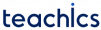# Full Subtractor

A full subtractor is a combinational circuit that performs subtraction of three bits. This circuit has three inputs the minuend A, subtrahend B, and borrow in Bin. Bin is the borrow of the previous adjacent lower minuend bit. This Bin is also subtracted from A-B. A full subtractor has two outputs, D and Bout, represents Difference and Borrow Out respectively.

Truth table of a full subtractor is,

A B Bin D Bout
00000
00111
01011
01101
10010
10100
11000
11111

The logic expression for full subtractor can be derived from the truth table using the K-Map technique.Expression for Bout can be again simplified as followsThese expressions can be implemented using two XOR gates, two AND gates and one OR gate as follows